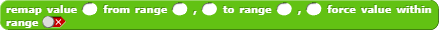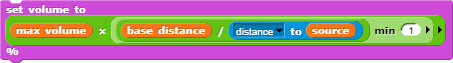# Making a sound increase based on distance

What's problem you are encountering?
I need a way to increase the volume of a sound depending on how close a sprite is to another sprite
What have you tried that didn't work?
The distance block reports the opposite and sounds can only be a max of 100% volume

I can’t test it with sounds because on I pads sound doesn’t work

100% minus distance?

Kind of, but It needs to be larger than just 100 units
And I can’t exactly test it

I think dividing it would work, but the issue is the distance can be larger than 100 so subtracting it also won’t

why not scaling it for example 200 meters could be 100%-(distance/2)

You need my REMAP block: see this thread

For your use case, remap the value "distance to" 0,500,100,0
(if the distance is 0 volume = 100, if the distance is 500 volume = 0, etc...)

Can you put that into block form?

Just saying, they don't NEED your remap block. The math is actually not that hard, once you understand it.

The math is just finding the percentage of the value in some other value, then putting that percentage inside another value. Here's what I mean, if you don't understand it.

But since you're just getting a percentage of 100, you don't even need the % of block.

Oh wait, you want it to be closer. The easy way to do that, just do 100 - result

Can you make that into an importable form?

It is, just click the image to make it big, then drag and drop it into snap.

Ohhhh

How do I change the distance

The 200 in the percentage of block is the number you want to change

surevalue: what value you want to remap

from range : define the interval of possible values you want to remap (distance in your case)(you can write 0,300 if you want...)

to range : the conversion range

(in this case when distance = 100, then the return value is 75 (the volume you want when distance = 100)

(in this case when distance = 300, then the return value is 0 (the volume you want when distance = 300)

let me know if you don't understand...

I don’t quite understand
What I’m making is a radar that gets louder depending on how far you are away from the target sprite, that sprite moves around, as well as getting faster

Can you share you project

It is Snap! Build Your Own Blocks
Sound blocks don’t work on apple that’s why I’m having issues

do you have another computer with which you can test the sound

In real life, a sound’s “intensity” (expressed in W/m2) is inversely proportional to the square of the distance from source to observer. E.g., if the intensity is 9 W/m^2 at 1 meter’s distance, at 2 meters it will be 9 / (2 x 2) = 2.25 W/m^2, and at 3 meters it will be 9 / (3 x 3) = 1 W/m^2.

What I don’t know is how that translates to values of Snap ! sound volume. It may be a logarithmical relationship, like with decibels: dV = 10 x log ( Ia / Ib)^2 = 20 x log ( Ia / Ib), with dV = difference in volume; Ia = intensity at distance a; Ib = intensity at distance b. If Snap ! volume percentage were to correspond to the same number of decibels, what it boils down to is the volume is changed by -6 every time the distance is doubled.

I don't think Snap ! sound volumes are in decibels, though.
I'd advise to divide the sound volume by two every time the distance doubles.
To make it all work you would need to specify:

1. a base distance, within which the sound is at its max, and
2. the maximum volume level itself.

TLDR use this block: